# Validate External Layer results with full Mesh#

This example displays post-processing comparisons between:
• results and mesh extracted only on external layer.

• results and mesh extracted on the entire mesh.

The external layer is the layer of solid elements with at least one facet facing the outside of the geometry. This feature is available for all types of Mechanical simulation, and allows you to reduce the size of the mesh and of the extracted data to improve processing performance. Since larger stress and strains are usually located on the skin of a model, computing the results on the external layer provides equivalent maximum values in most cases.

## Perform required imports#

This example uses a supplied file that you can get using the `examples` module.

```from ansys.dpf import post
from ansys.dpf.post import examples
```

## Get `Simulation` object#

Get the `Simulation` object that allows access to the result. The `Simulation` object must be instantiated with the path for the result file. For example, `"C:/Users/user/my_result.rst"` on Windows or `"/home/user/my_result.rst"` on Linux.

```example_path = examples.download_crankshaft()
# to automatically detect the simulation type, use:

# to enable auto-completion, use the equivalent:
simulation = post.StaticMechanicalSimulation(example_path)

# print the simulation to get an overview of what's available
print(simulation)
```
```Static Mechanical Simulation.

Data Sources
------------------------------
/opt/hostedtoolcache/Python/3.8.17/x64/lib/python3.8/site-packages/ansys/dpf/core/examples/result_files/crankshaft/crankshaft.rst

DPF Model
------------------------------
Static analysis
Unit system: MKS: m, kg, N, s, V, A, degC
Physics Type: Mechanical
Available results:
-  displacement: Nodal Displacement
-  velocity: Nodal Velocity
-  acceleration: Nodal Acceleration
-  reaction_force: Nodal Force
-  stress: ElementalNodal Stress
-  elemental_volume: Elemental Volume
-  stiffness_matrix_energy: Elemental Energy-stiffness matrix
-  artificial_hourglass_energy: Elemental Hourglass Energy
-  thermal_dissipation_energy: Elemental thermal dissipation energy
-  kinetic_energy: Elemental Kinetic Energy
-  co_energy: Elemental co-energy
-  incremental_energy: Elemental incremental energy
-  elastic_strain: ElementalNodal Strain
-  structural_temperature: ElementalNodal Structural temperature
------------------------------
DPF  Meshed Region:
69762 nodes
39315 elements
Unit: m
With solid (3D) elements
------------------------------
DPF  Time/Freq Support:
Number of sets: 3
1              1.000000       1              1
2              2.000000       1              2
3              3.000000       1              3
```

## Extract displacement data#

Extract displacement data over the entire mesh and on the external layer.

```displacement_ext = simulation.displacement(external_layer=True)
displacement = simulation.displacement()  # default is external_layer=False
displacement_ext.plot()
displacement.plot()

print(
f"number of nodes with external_layer=True: {len(displacement_ext.index.mesh_index)}"
)
print(
f"number of nodes with external_layer=False: {len(displacement.index.mesh_index)}"
)
```
•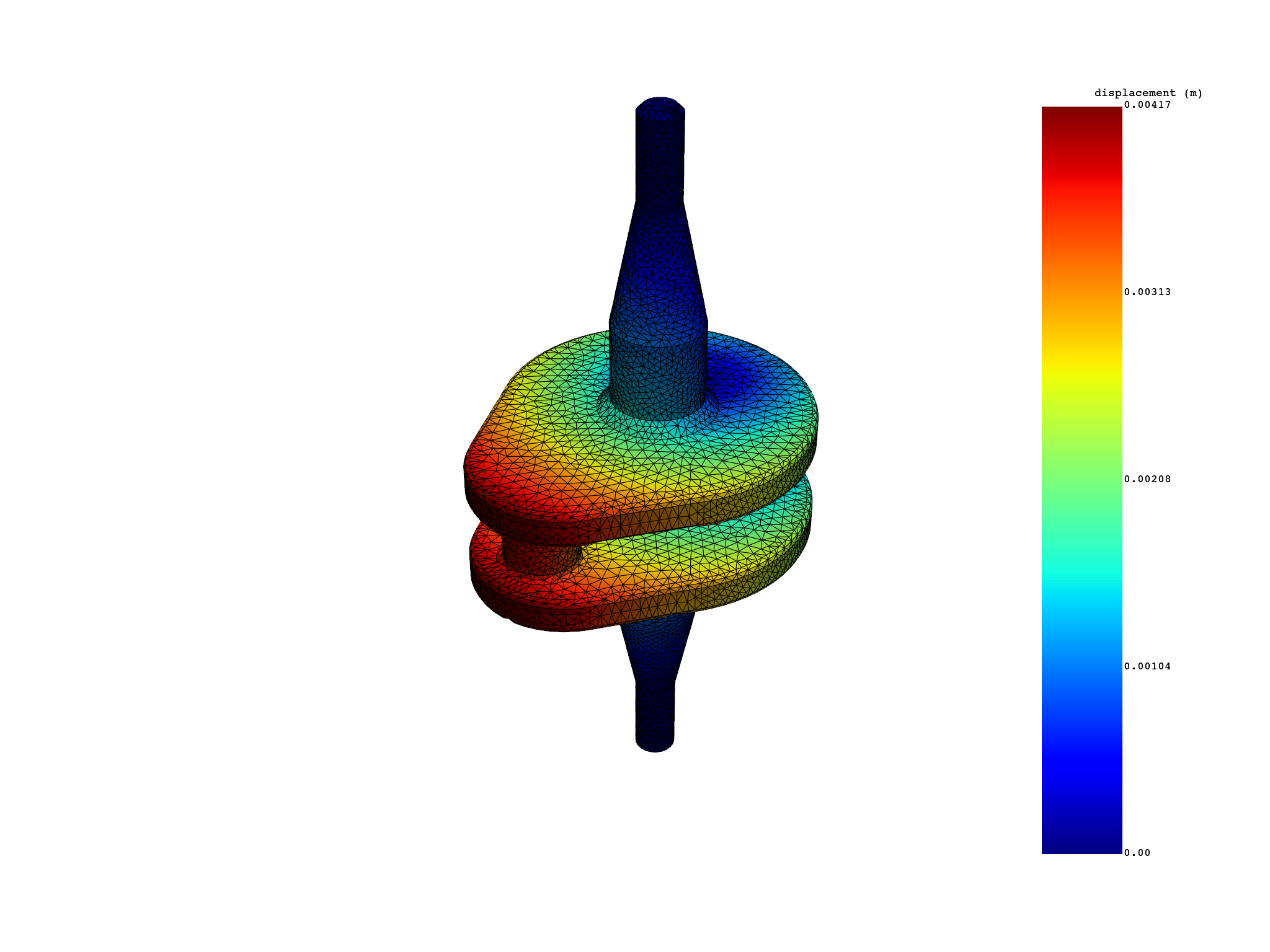•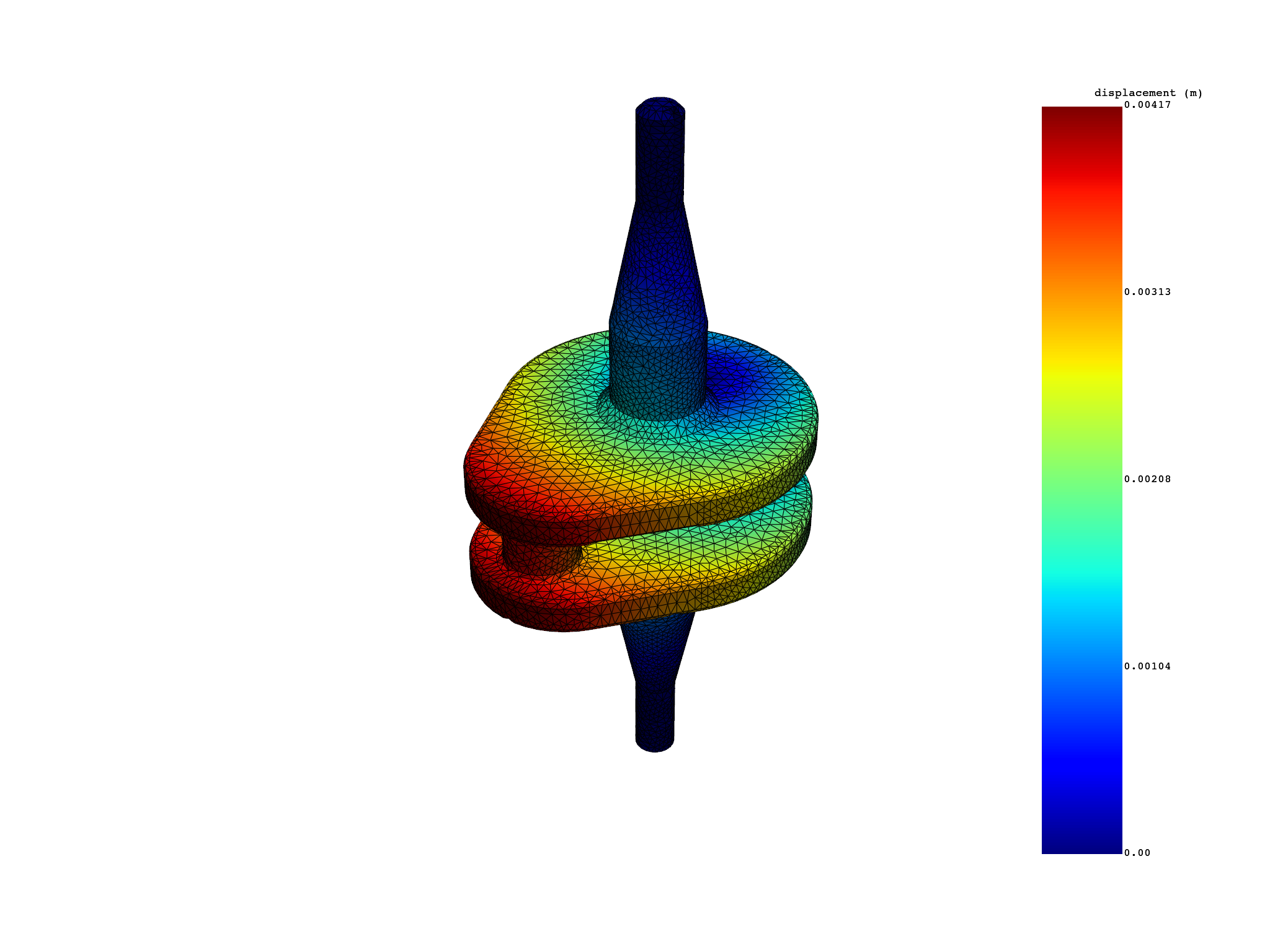```number of nodes with external_layer=True: 64079
number of nodes with external_layer=False: 69762
```

## Extract stress/strain data#

Extract stress or elastic strain data over the entire mesh and on the external layer. Averaging, and invariants computation can easily be done on the external layer since the connectivity of the kept elements remains unchanged.

```elemental_stress_ext = simulation.stress_principal_elemental(
components=, external_layer=True
)
elemental_stress = simulation.stress_principal_elemental()
elemental_stress_ext.plot()
elemental_stress.plot()

print(
f"number of elements with external_layer=True: {len(elemental_stress_ext.index.mesh_index)}"
)
print(
f"number of elements with external_layer=False: {len(elemental_stress.index.mesh_index)}"
)

elastic_strain_eqv_ext = simulation.elastic_strain_eqv_von_mises_nodal(
external_layer=True
)
elastic_strain_eqv = simulation.elastic_strain_eqv_von_mises_nodal()
elastic_strain_eqv_ext.plot()
elastic_strain_eqv.plot()
```
•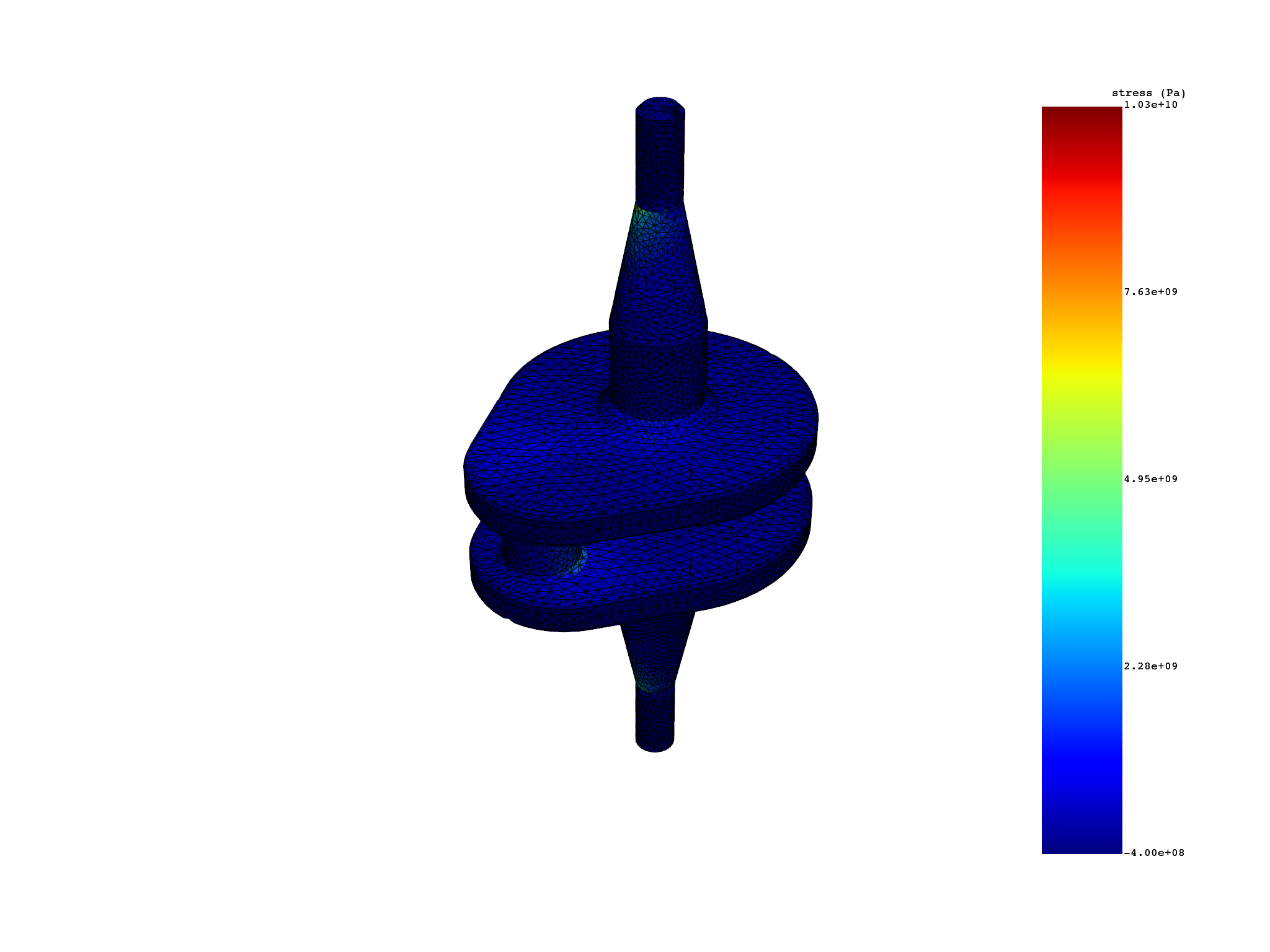•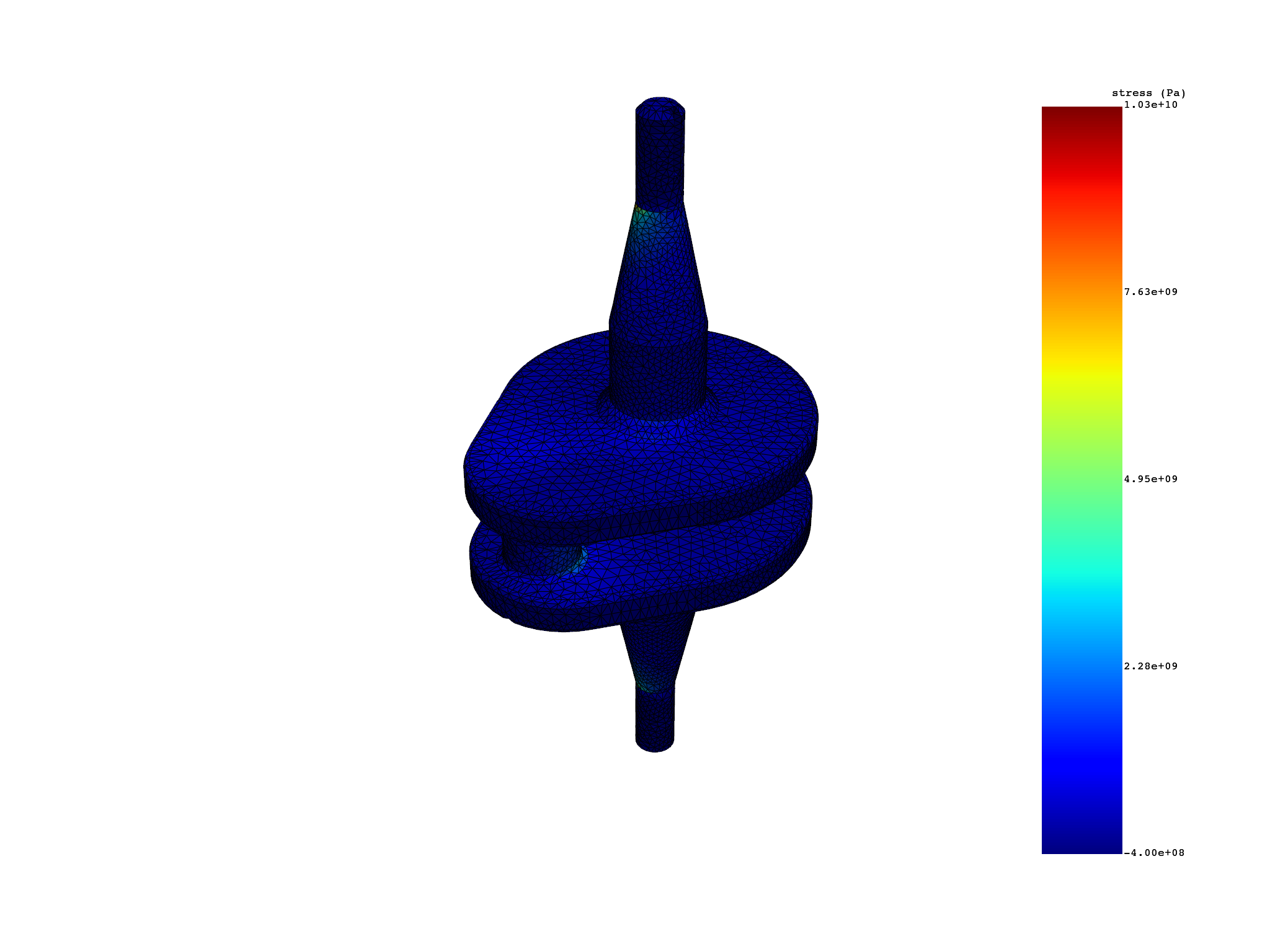•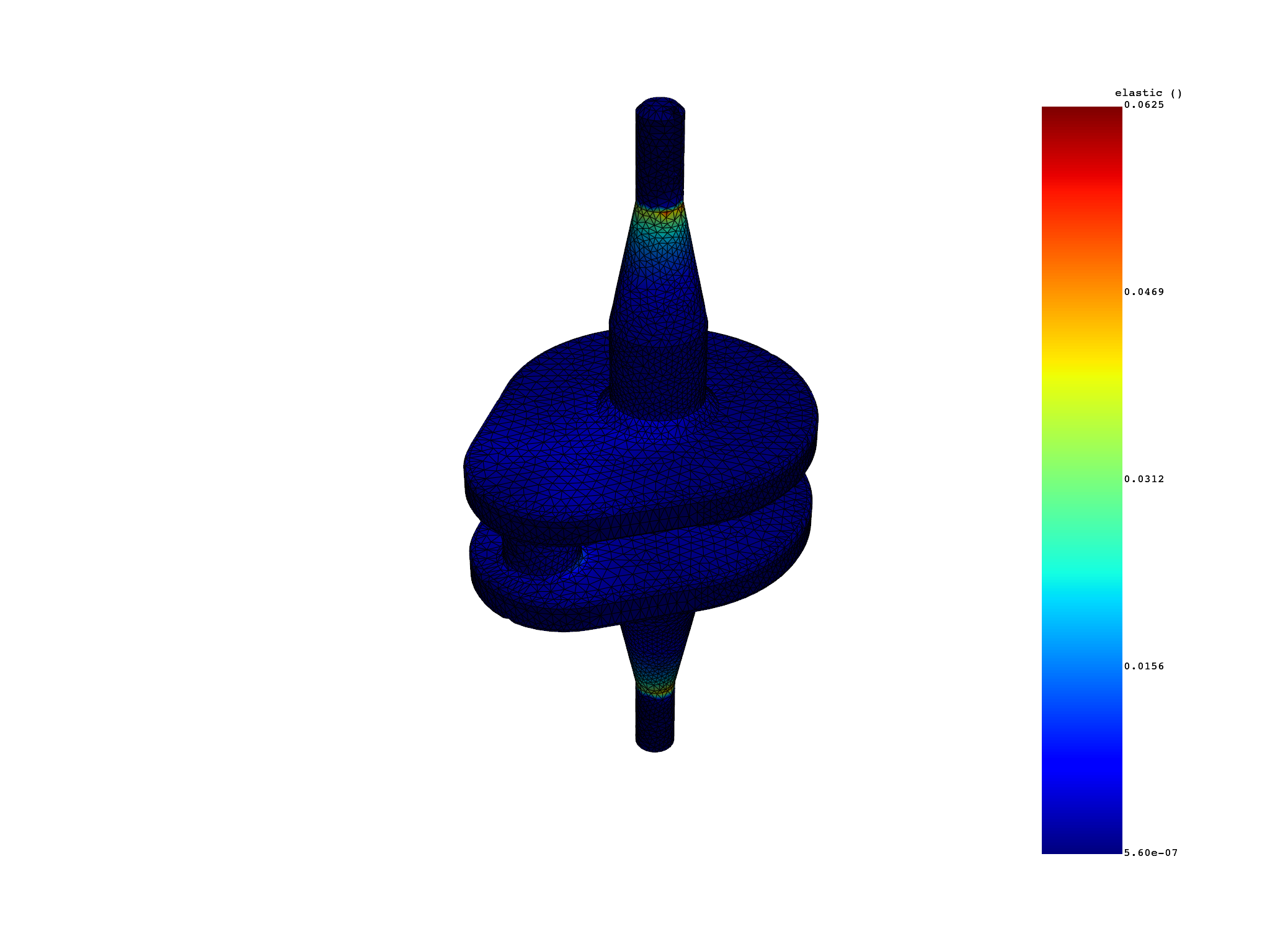•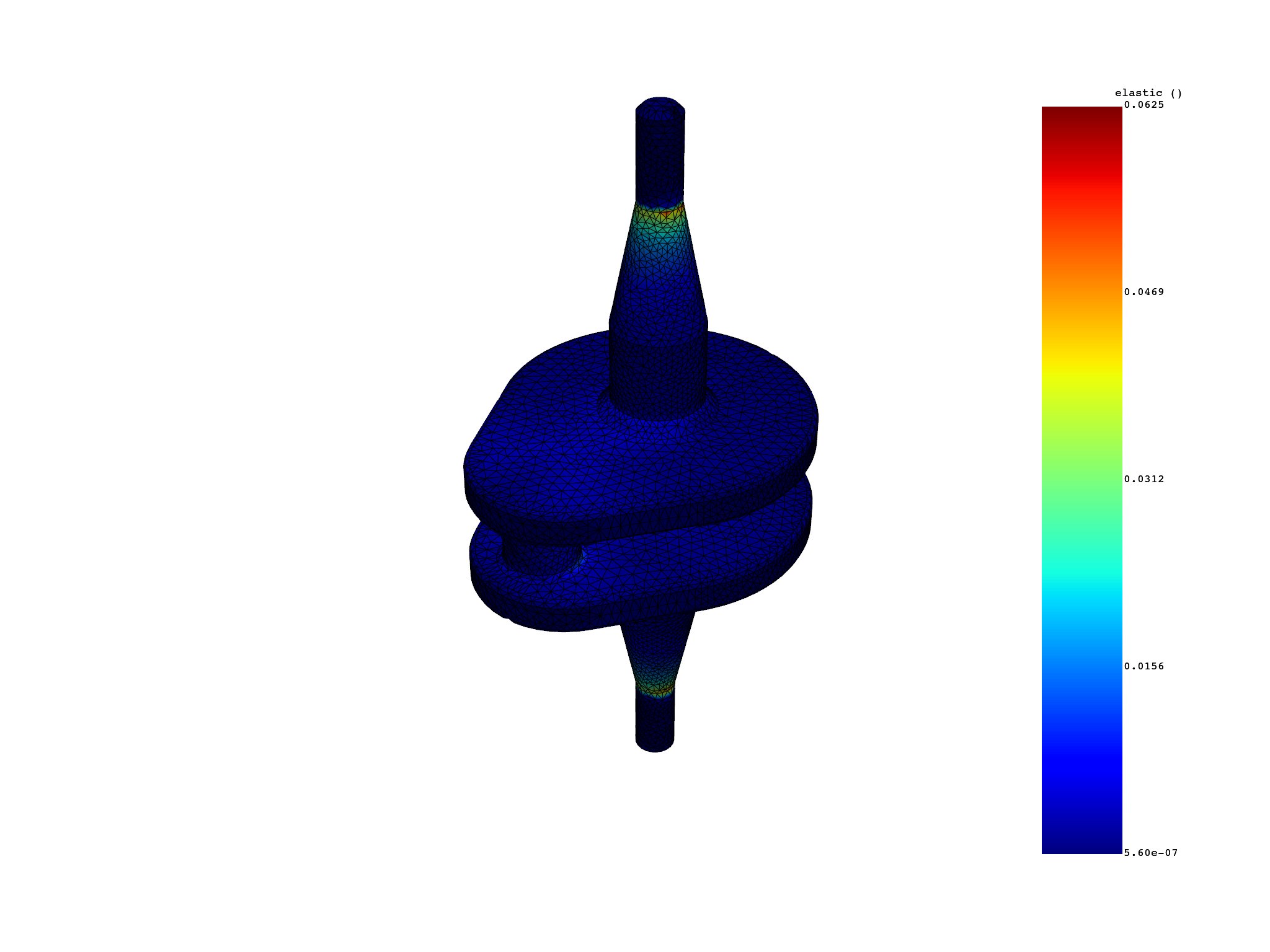```number of elements with external_layer=True: 31374
number of elements with external_layer=False: 39315
```

Total running time of the script: ( 0 minutes 5.041 seconds)

Gallery generated by Sphinx-Gallery